# Python Reverse String: The Complete Guide

0
69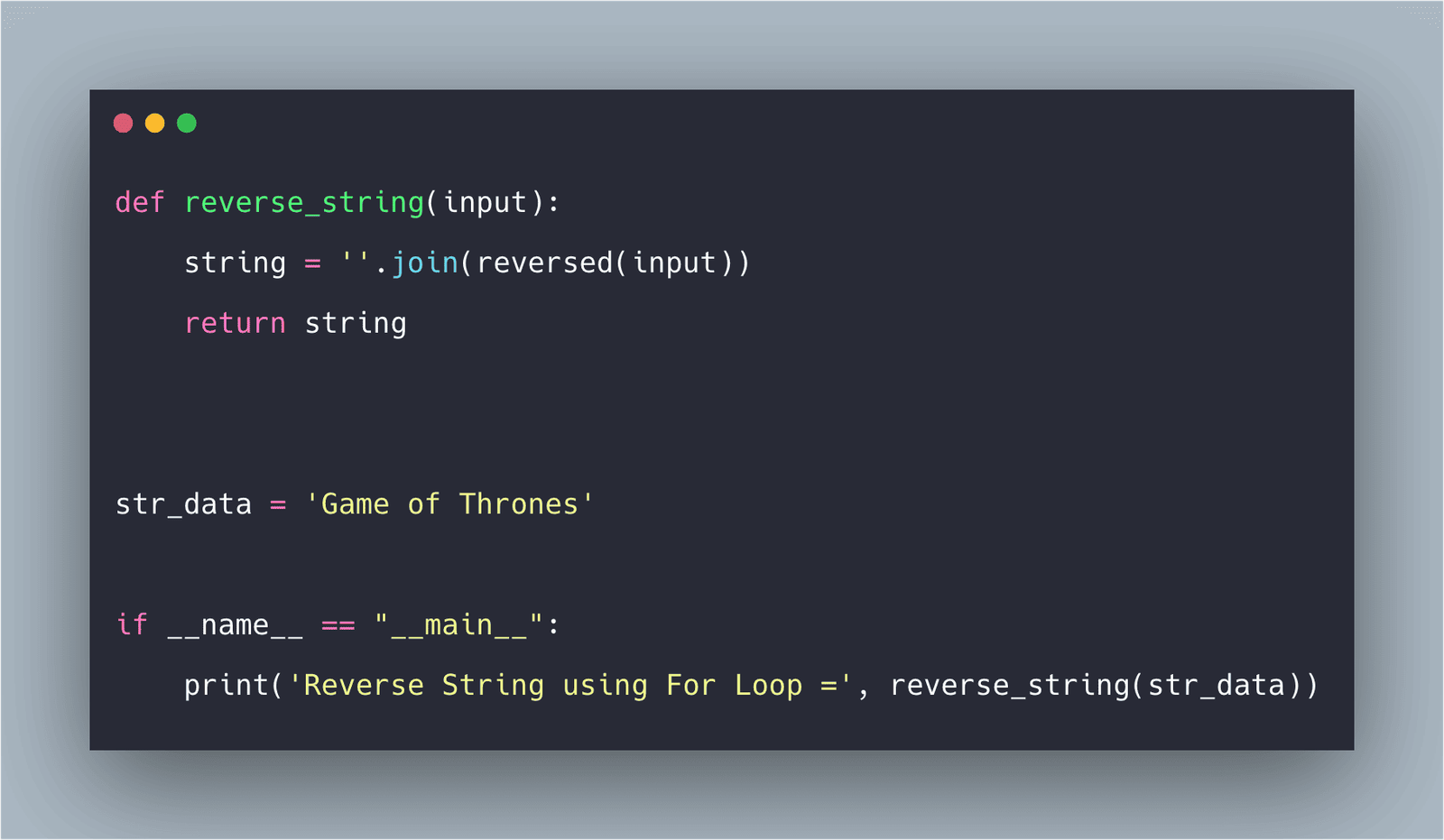Python doesn’t have a built-in reverse() function. However, there are various ways to reverse any string in Python. The fastest (and easiest?) way is to use a slice that steps backward, -1. Python String reversal isn’t used often in day-to-day programming, but it’s still a popular interviewing question.

## How to Reverse String In Python

To reverse a string in Python, some of the common ways to reverse string are the following.

1. Using Slicing to create a reverse copy of the string.
2. Using for loop and appending characters in reverse order.
3. Using while loop to iterate string characters in reverse order and append them.
4. Using string join() function with reversed() iterator.
5. Creating a list from the string and then calling its reverse() function.
6. Using Recursion.

See the following example.

```name = 'AppDividend'

reversedName = 'dnediviDppA'```

In the above code, the first name variable is a standard variable, and then reversedName is the reverse string.

## Reversing a Python String With the Slicing

Strings follow the sequence protocol in Python. And all sequences support an exciting feature called slicing. You can view slicing as the extension of the square-brackets indexing syntax.

It includes a particular case where slicing the sequence with “[::-1]” produces the reversed copy of the string. Because Python strings are sequences, this is the quick and easy way to get a reversed copy of the string.

See the following code example.

```# app.py

name = 'AppDividend'
reversedName = name[::-1]
print(reversedName)```

See the output.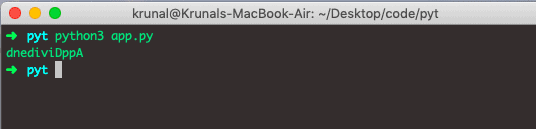It’s short and sweet—but, in my mind, but the downside to reversing the string with a slicing syntax is that it uses the advanced Python feature that some developers would say is “arcane.”

My biggest gotcha here is that it uses “[::-1]” slicing syntax doesn’t communicate enough that it creates the reversed copy of the original string.

See the second example of Python Reverse String using Slicing.

```# app.py

def reverse_string(str):
return str[::-1]

str_data = 'Game of Thrones'

if __name__ == "__main__":
print('Reverse String using Python slicing =', reverse_string(str_data))```

See the below output.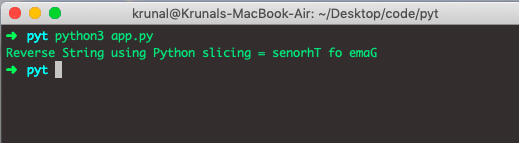## Using a loop to reverse the string

In the below example, we are reversing the string using for loop.

```# app.py

def reverse_string(str):
string = ""
for i in str:
string = i + string
return string

str_data = 'Game of Thrones'

if __name__ == "__main__":
print('Reverse String using For Loop =', reverse_string(str_data))```

See the below output.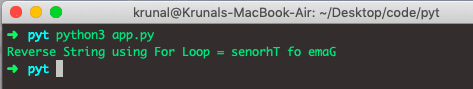## Python Reverse String using While Loop

See the following code.

```# app.py

def reverse_string(input):
string = ""
length = len(input) - 1
while length >= 0:
string = string + input[length]
length = length - 1
return string

str_data = 'Game of Thrones'

if __name__ == "__main__":
print('Reverse String using While Loop =', reverse_string(str_data))```

## Python reverse string using join() and reversed()

Reversing the string using reverse iteration with the built-in is another option. You get the reverse iterator you can use to cycle through the elements in the string in reverse order.

Using the reversed() function does not modify an original string (which wouldn’t work anyway as strings are the immutable objects in Python.) What happens is that you get the “view” into the existing string you can use to look at all the items in reverse order.

Now, We used Python join() function and reversed() function combined to get the reversed string. See the below example.

```# app.py

def reverse_string(input):
string = ''.join(reversed(input))
return string

str_data = 'Game of Thrones'

if __name__ == "__main__":
print('Reverse String using For Loop =', reverse_string(str_data))
```

The above code snippet used the .join() method to merge all of the string characters resulting from the reversed iteration into the new string.

Now, see the output.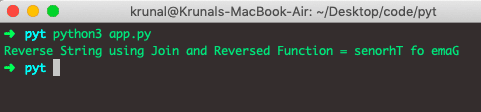I like the above reverse iterator approach for reversing the strings in Python.

It communicates clearly what is going on, and even someone new to the language would eventually understand that I’m creating the reversed copy of an original string.

And while understanding how iterators work at a deeper level is helpful, it’s not necessary to use this technique.

## Python Reverse String using Recursion

See the below code.

```# app.py

def reverse_string(input):
if len(input) == 0:
return input
else:
return reverse_string(input[1:]) + input

str_data = 'Game of Thrones'

if __name__ == "__main__":
print('Reverse String using Recursion =', reverse_string(str_data))```

Now, see the output.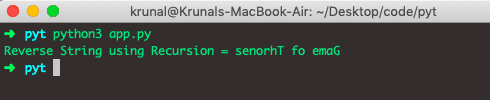## Performance Comparison

After implementing a string reversal approach, I have shown you many approaches in this tutorial and now see their relative performance.

Slicing is the fastest approach, the reversed() approach is 8x slower than slicing and then while and for loop also takes some time.

If you’re wondering which the best way is to reverse the string in Python, my answer is: “It depends.”

However, there’s an argument to be made that the eight times faster slicing approach should be definitely used for reasons of performance. The performance cost is always a good parameter on which you should build your web application. That is it for this example.

That’s it for this tutorial.

This site uses Akismet to reduce spam. Learn how your comment data is processed.